Explaining model using SHAP¶

View the graphs using this link: https://nbviewer.jupyter.org/github/chaitanyakasaraneni/SHAP_EBM_Examples/blob/main/SHAP%20Example.ipynb

In :
import pandas as pd
import numpy as np
import plotly
np.random.seed(0)
import matplotlib.pyplot as plt
In :
In :
df.shape
Out:
(1599, 12)
In :
df.columns
df['quality'] = df['quality'].astype(int)
In :
Out:
fixed acidity volatile acidity citric acid residual sugar chlorides free sulfur dioxide total sulfur dioxide density pH sulphates alcohol quality
0 7.4 0.70 0.00 1.9 0.076 11.0 34.0 0.9978 3.51 0.56 9.4 5
1 7.8 0.88 0.00 2.6 0.098 25.0 67.0 0.9968 3.20 0.68 9.8 5
2 7.8 0.76 0.04 2.3 0.092 15.0 54.0 0.9970 3.26 0.65 9.8 5
3 11.2 0.28 0.56 1.9 0.075 17.0 60.0 0.9980 3.16 0.58 9.8 6
4 7.4 0.70 0.00 1.9 0.076 11.0 34.0 0.9978 3.51 0.56 9.4 5
In :
df['quality'].hist()
Out:
<AxesSubplot:>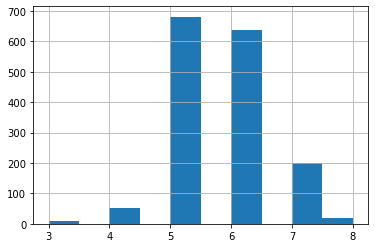In :
from sklearn.model_selection import train_test_split
from sklearn import preprocessing
from sklearn.ensemble import RandomForestRegressor

Y = df['quality']
X =  df[['fixed acidity', 'volatile acidity', 'citric acid', 'residual sugar',
'chlorides', 'free sulfur dioxide', 'total sulfur dioxide', 'density',
'pH', 'sulphates', 'alcohol']]
In :
X_train, X_test, Y_train, Y_test = train_test_split(X, Y, test_size = 0.25)
In :
model = RandomForestRegressor(max_depth=6, random_state=0, n_estimators=10)
model.fit(X_train, Y_train)
Out:
RandomForestRegressor(max_depth=6, n_estimators=10, random_state=0)

Feature Importance using SHAP - Global Interpretability¶

In :
import shap
In :
shap_values = shap.TreeExplainer(model).shap_values(X_train)
In :
shap.summary_plot(shap_values, X_train, plot_type="bar")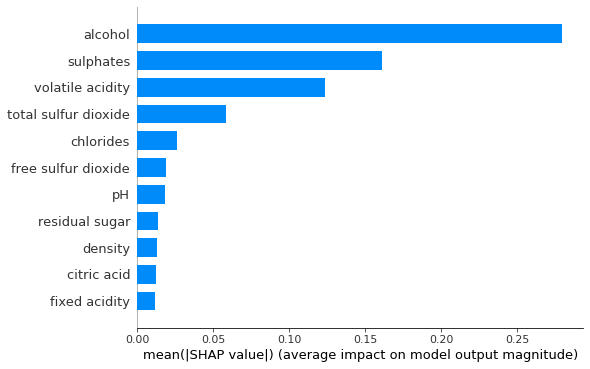In :
shap.summary_plot(shap_values, X_train)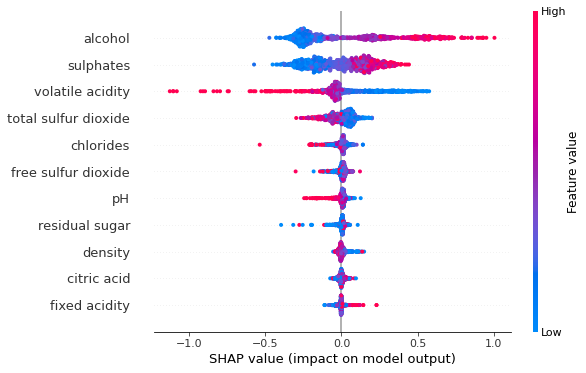Based on the graph, high quality rating of wine is associated with the following characteristics:

• High alcohol content
• High sulphates
• Low volatile acidity
• Low total sulfuer dioxide
• Low pH
• Low chlorides
• Low citric acid
• Low density
• High fixed acidity content
• High free sulfur dioxide
• High residual sugar

Effects of Single Feature on the output - All Data (Global Interpretability)¶

The partial dependence plot shows the marginal effect one or two features have on the predicted outcome of a machine learning model. It tells whether the relationship between the target and a feature is linear, monotonic or more complex. In order to create a dependence plot, you only need one line of code:

shap.dependence_plot("alcohol", shap_values, X_train)

The function automatically includes another variable that your chosen variable interacts most with.

In :
shap.dependence_plot("alcohol", shap_values, X_train)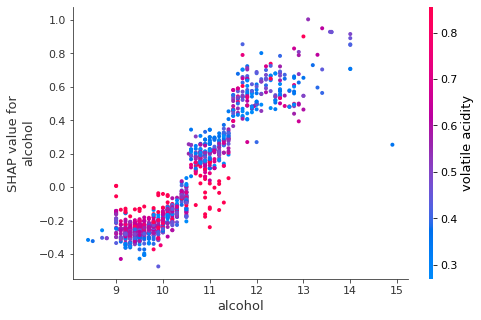In :
shap.dependence_plot("volatile acidity", shap_values, X_train)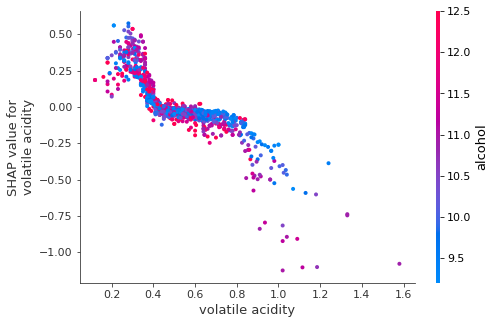In :
shap.dependence_plot("total sulfur dioxide", shap_values, X_train)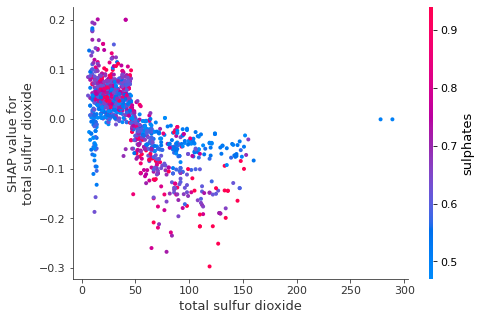Effects of Single Feature on the output - Each Input Data (Local Interpretability)¶

Remember the SHAP model is built on the training data set. The means of the variables are:

In :
X_train.mean()
Out:
fixed acidity            8.338032
volatile acidity         0.530388
citric acid              0.271034
residual sugar           2.557882
chlorides                0.087227
free sulfur dioxide     16.132611
total sulfur dioxide    47.371977
density                  0.996757
pH                       3.308874
sulphates                0.658757
alcohol                 10.405922
dtype: float64
In :
# Get the predictions and put them with the test data.
X_output = X_test.copy()
X_output.loc[:,'predict'] = np.round(model.predict(X_output),2)

# Randomly pick some observations
random_picks = np.arange(1,330,50)
S = X_output.iloc[random_picks]
S
Out:
fixed acidity volatile acidity citric acid residual sugar chlorides free sulfur dioxide total sulfur dioxide density pH sulphates alcohol predict
1032 8.1 0.820 0.00 4.1 0.095 5.0 14.0 0.99854 3.36 0.53 9.6 4.77
34 5.2 0.320 0.25 1.8 0.103 13.0 50.0 0.99570 3.38 0.55 9.2 5.15
1508 7.1 0.270 0.60 2.1 0.074 17.0 25.0 0.99814 3.38 0.72 10.6 6.60
1479 8.2 0.280 0.60 3.0 0.104 10.0 22.0 0.99828 3.39 0.68 10.6 6.00
866 6.8 0.490 0.22 2.3 0.071 13.0 24.0 0.99438 3.41 0.83 11.3 6.24
1519 6.6 0.700 0.08 2.6 0.106 14.0 27.0 0.99665 3.44 0.58 10.2 5.47
1193 6.4 0.885 0.00 2.3 0.166 6.0 12.0 0.99551 3.56 0.51 10.8 4.82
In :
# Initialize your Jupyter notebook with initjs(), otherwise you will get an error message.
shap.initjs()

def shap_plot(j):
explainerModel = shap.TreeExplainer(model)
shap_values_Model = explainerModel.shap_values(S)
p = shap.force_plot(explainerModel.expected_value, shap_values_Model[j], S.iloc[[j]])
return(p)In :
shap_plot(0)
Out:
Visualization omitted, Javascript library not loaded!
Have you run initjs() in this notebook? If this notebook was from another user you must also trust this notebook (File -> Trust notebook). If you are viewing this notebook on github the Javascript has been stripped for security. If you are using JupyterLab this error is because a JupyterLab extension has not yet been written.

Explaining the plot for First Row in the above table¶

• The output value is the prediction for that particular observation i.e., the graph above corresponds to the first row in the table above where the predicted output is 4.77
• Red/blue: Features that push the prediction higher (to the right) are shown in red, and those pushing the prediction lower are in blue.
• Alcohol: has a positive impact on the quality rating. The alcohol content of this wine is 9.6 (as shown in the first row of Table) which is lower than the average value 10.406. So it pushes the prediction to the left.
• pH: has a negative impact on the quality rating. A higher than the average pH (= 3.36 > 3.30) drives the prediction to the left.
• Sulphates: is positively related to the quality rating. A lower than the average Sulphates (= 0.53 < 0.65) pushes the prediction to the left.

Similarly, the following are individual graphs for data rows 2,3,4, and 5

In :
shap_plot(1)
Out:
Visualization omitted, Javascript library not loaded!
Have you run initjs() in this notebook? If this notebook was from another user you must also trust this notebook (File -> Trust notebook). If you are viewing this notebook on github the Javascript has been stripped for security. If you are using JupyterLab this error is because a JupyterLab extension has not yet been written.
In :
shap_plot(2)
Out:
Visualization omitted, Javascript library not loaded!
Have you run initjs() in this notebook? If this notebook was from another user you must also trust this notebook (File -> Trust notebook). If you are viewing this notebook on github the Javascript has been stripped for security. If you are using JupyterLab this error is because a JupyterLab extension has not yet been written.
In :
shap_plot(3)
Out:
Visualization omitted, Javascript library not loaded!
Have you run initjs() in this notebook? If this notebook was from another user you must also trust this notebook (File -> Trust notebook). If you are viewing this notebook on github the Javascript has been stripped for security. If you are using JupyterLab this error is because a JupyterLab extension has not yet been written.
In :
shap_plot(4)
Out:
Visualization omitted, Javascript library not loaded!
Have you run initjs() in this notebook? If this notebook was from another user you must also trust this notebook (File -> Trust notebook). If you are viewing this notebook on github the Javascript has been stripped for security. If you are using JupyterLab this error is because a JupyterLab extension has not yet been written.

SHAP interaction values¶

In :
shap_interaction_values = shap.TreeExplainer(model).shap_interaction_values(X_train.iloc[:2000,:])

shap.summary_plot(shap_interaction_values, X_train.iloc[:2000,:])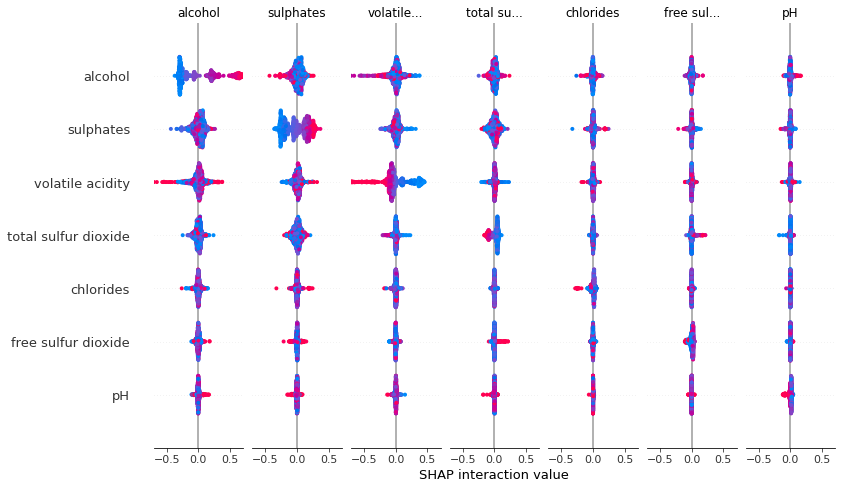In [ ]: